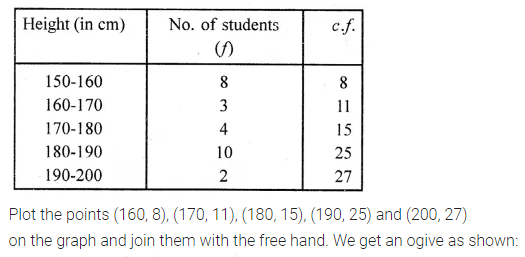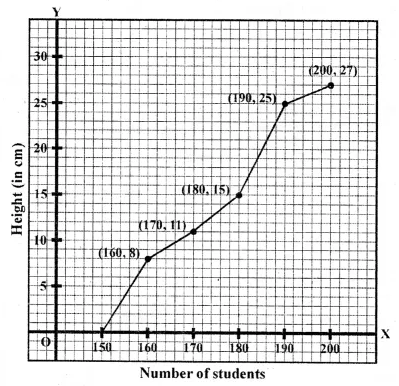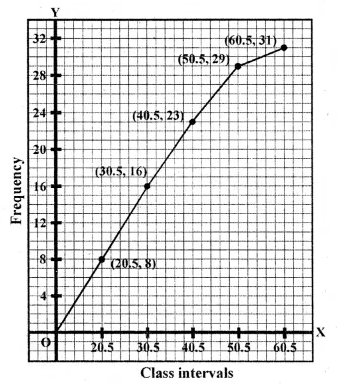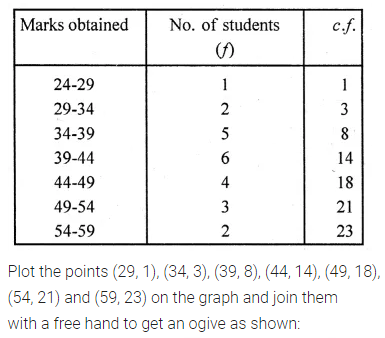# ML Aggarwal Class 10 Solutions for ICSE Maths Chapter 21 Measures of Central Tendency Ex 21.5

## ML Aggarwal Class 10 Solutions for ICSE Maths Chapter 21 Measures of Central Tendency Ex 21.5

ML Aggarwal Class 10 Solutions for ICSE Maths Chapter 21 Measures of Central Tendency Ex 21.5

Question 1.
Draw an ogive for the following frequency distribution:Solution:Question 2.
Draw an ogive for the following data:Solution:Question 3.
Draw a cumulative frequency curve for the following data:Solution: